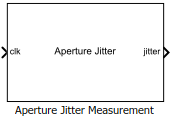# Aperture Jitter Measurement

Measure aperture jitter of periodic signals

• Library:
• Mixed-Signal Blockset / ADC / Measurements & Testbenches

•## Description

The Aperture Jitter Measurement measure the aperture jitter of periodic signals. In practical data converters, there is a delay between the sampling edge of the sample clock signal and when the sample is actually taken. This delay is known as aperture delay. Aperture jitter is the sample to sample variation between aperture delay.

## Ports

### Input

expand all

Clock signal input, specified as a scalar in volts.

Data Types: `double`

### Output

expand all

Aperture jitter output, returned as a scalar in seconds.

Data Types: `double`

## Parameters

expand all

Input clock frequency, specified as a real positive scalar in Hz.

#### Programmatic Use

• Use `get_param(gcb,'Frequency')` to view the current value of Frequency.

• Use `set_param(gcb,'Frequency',value)` to set Frequency to a specific value.

Minimum time the simulation must run for meaningful result, specified as a scalar in seconds. This is calculated using the JEDEC Standards for measuring aperture jitter.

Signal range

Minimum value of clock signal, specified as a real scalar in volts.

#### Programmatic Use

• Use `get_param(gcb,'InputMin')` to view the current value of Min.

• Use `set_param(gcb,'InputMin',value)` to set Min to a specific value.

Maximum value of clock signal, specified as a real scalar in volts.

#### Programmatic Use

• Use `get_param(gcb,'InputMax')` to view the current value of Max.

• Use `set_param(gcb,'InputMax',value)` to set Max to a specific value.

Delays measurement analysis to avoid corruption by transients, specified as a nonnegative real scalar in seconds.

#### Programmatic Use

• Use `get_param(gcb,'HoldOffTime')` to view the current value of Hold off time (s).

• Use `set_param(gcb,'HoldOffTime',value)` to set Hold off time (s) to a specific value.

Data Types: `double`

## Version History

Introduced in R2019a